# The correlation matrix and the variance of a portfolio

4.4Let’s pick up from right where we left off. Towards the end of the previous chapter, we set up the variance-covariance matrix for stock A, stock B and stock C. This is what it looked like.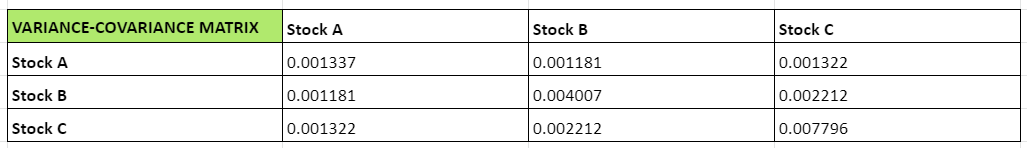This will be the basis for calculating the risk in the portfolio. In other words, the portfolio variance. To get there, we need to calculate a few things.

• The standard deviation of each stock in the portfolio
• The correlation matrix
• The weighted standard deviation of each stock in the portfolio

We’ll take this up one by one.

### The standard deviation of each stock in the portfolio

We can use excel to calculate this easily, using the standard deviation function.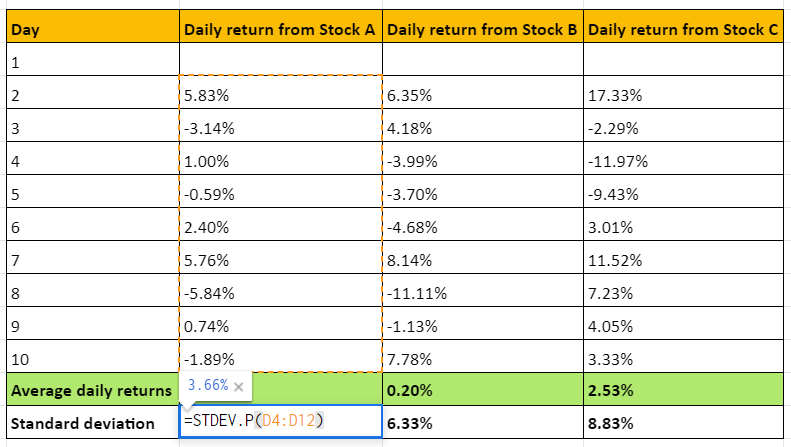So, we get the following standard deviations:

• Stock A: 3.66%
• Stock B: 6.33%
• Stock C: 8.83%

We’ll put this in a column matrix, so our work becomes easier. Here it is.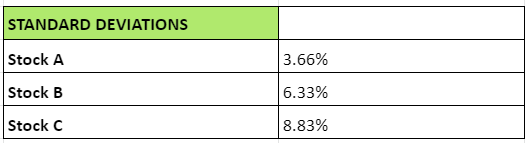### The correlation matrix

To calculate the correlation between two stocks, say stock A and stock B, we use this formula:

 Correlation between stock A and stock B = Covariance (A, B) ÷ (SDA x SDB)
• Here, the covariance of two stocks can easily be taken from the variance-covariance matrix.
• SDA and SDB represent the standard deviations of the stocks. So, basically, we need to find the products of standard deviations of each pair of stocks in the portfolio.

For this, we’ll need matrices once more. We can calculate the standard deviation products of each pair of stocks by multiplying the column matrix shown above with its transpose. This is how it looks.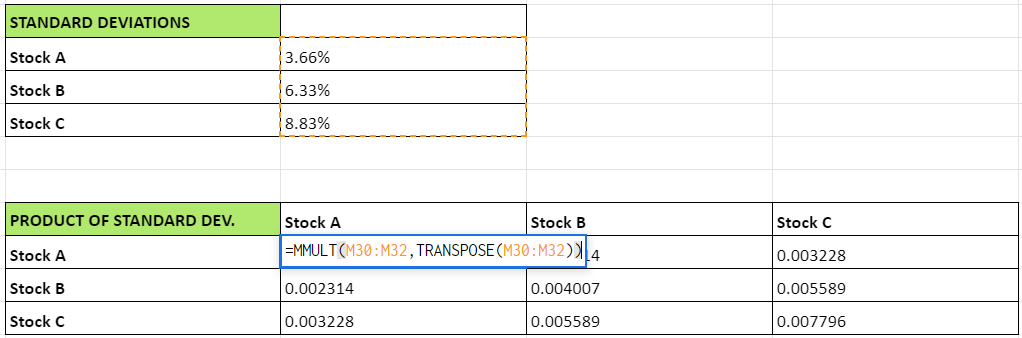The matrix with the products of standard deviation is here: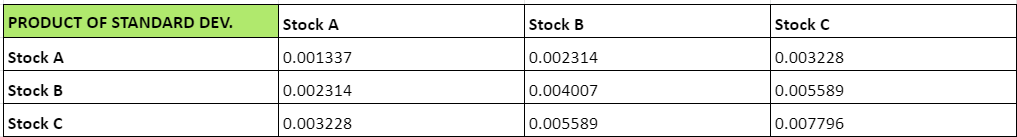So, we now have everything to calculate the correlation, which is basically the covariance of two stocks divided by the product of its standard deviations.

• The numerator for this is the covariance, which we’ll take from the variance-covariance matrix.
• The denominator is available in the ‘product of the SDs’ matrix, as seen in the image above.
 NumeratorDenominatorUsing excel, this is the correlation matrix we get: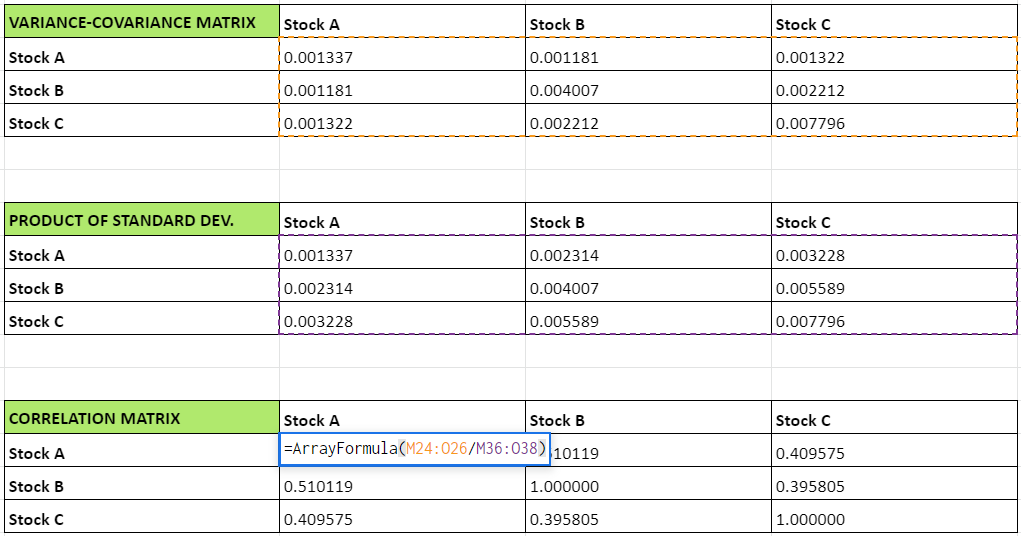### Understanding the correlation matrix

This is how the correlation matrix looks for the three stocks.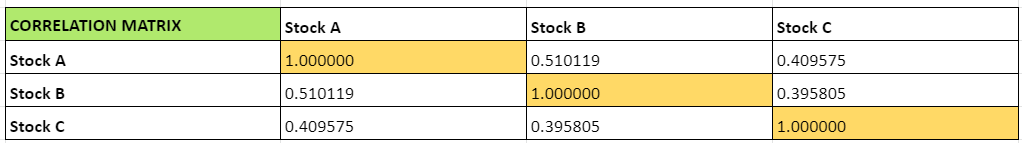What is the correlation matrix and what does it tell you? Simply put, it gives you the correlation between two stocks. Remember we discussed it in the beginning of this module? The correlation ranges from -1 to +1 and shows how strongly two stocks are related to one another. The higher the number, the stronger the correlation between two stocks.

Here, the cells shown in yellow represent the correlation between stock A and itself, stock B and itself, and stock C in itself. Naturally, this will be the highest possible, which is why it is +1.

Meanwhile, check out the correlation between stock A and stock C, and stock C and stock A. They are both 0.409575. The correlation between these two stocks will be the same whichever way you look at it.

With this done, it’s time to enter the last leg of determining the risk of a portfolio - the calculator of portfolio variance.

### The variance of the portfolio

To find out the variance of a portfolio, we’ll need the weighted standard deviations of the stocks in it. So, let’s assign arbitrary weights to the stocks in the portfolio. Weights here refer to the percentage of overall capital invested in that stock.

We’re just going to do this randomly for now. Later in this module, we’ll be looking at the science behind it.

So, here it goes.

• Stock A: 20%
• Stock B: 35%
• Stock C: 45%

The weighted standard deviation is simply the product of the weights assigned above, and the standard deviation of each stock.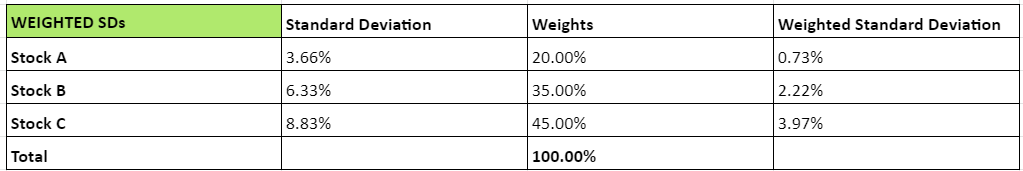### The formula for portfolio variance

To calculate the portfolio variance, this is the formula we’re going to use.

 Portfolio variance [Transpose of the weighted SD matrix * Correlation matrix * Weighted SD]1/2

So, here’s what we need to do, step-by-step.

1. Create the transpose of the weighted SD matrix
2. Multiply the above matrix with the correlation matrix
3. Multiply the product from the previous step with the weighted SD
4. Find the square root of the product obtained in the previous step

### Step 1: Create the transpose of the weighted SD matrix

Weighted SD matrix:

This is a column matrix with a size of 3 x 1.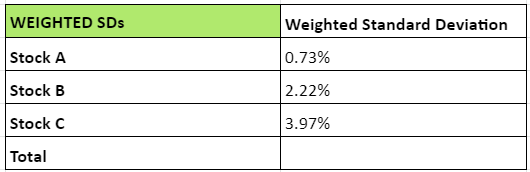Transpose of the weighted SD matrix:

The transpose of the column matrix above will naturally be a row matrix with the size of 1 x 3, as follows: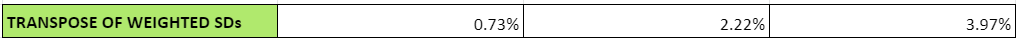### Step 2: Multiply the transpose matrix above with the correlation matrix

• The transpose matrix seen above is a 1 x 3 matrix.
• The correlation matrix we obtained earlier is a 3 x 3 matrix.
• So, the number of columns in the first matrix matches the number of rows in the second matrix. This makes multiplication possible.
• The resulting matrix will be a 1 x matrix, as follows. We’ll call this matrix as V1, for the sake of easier referencing.

Matrix V1 = Transpose of weighted SD matrix * Correlation matrix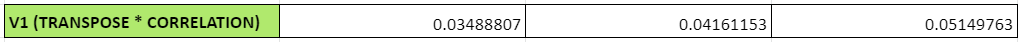### Step 3: Multiply the matrix from the previous step (V1) with the weighted SD

The weighted SD matrix is essentially a 3 x 1 column matrix.We have to multiply matrix V1, with dimensions of 1 x 3, with this weighted SD matrix of 3 x 1. The resulting matrix - we’ll call that V2 - will have dimensions of 1 x 1. Essentially, that will be a single number. Interesting, right? Check it out below.

Matrix V2 = Matrix V1 * Weighted SD matrix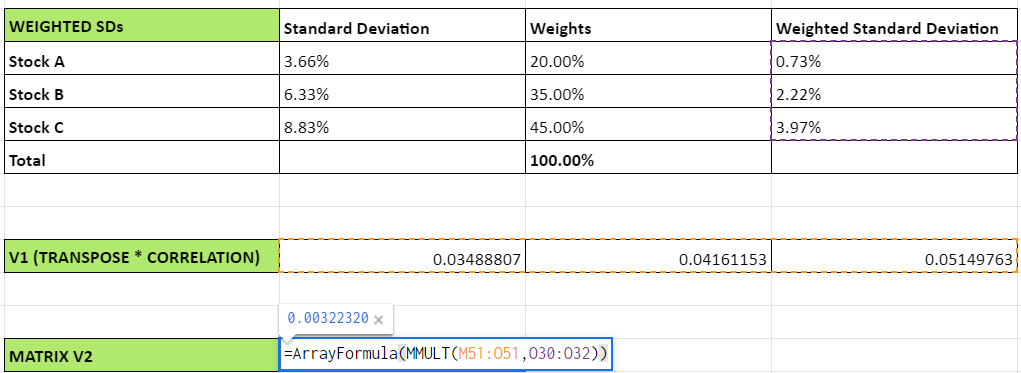So, V2 is a single number: 0.00322320

### Step 4: Find the square root of the product obtained in the previous step (V2)

The square root of 0.00322320 comes out to be 0.05677320. Or, in other words, 5.68%. This is the portfolio variance.

#### Wrapping up

This is the daily variance of our three-stock portfolio. So, on any given day, you can expect a variance of 5.68%. This corresponds to 1 SD, as per the normal distribution pattern. By extension, 2 SD would be 11.36% and 3 SD would be 17.04%. So, on a daily basis, you can expect the following variations with the mentioned levels of certainty.

• The returns will vary by 5.68% on either side of the average expected returns of the portfolio, with a 68% certainty.
• The returns will vary by 11.36% on either side of the average expected returns of the portfolio, with a 95% certainty.
• The returns will vary by 17.04% on either side of the average expected returns of the portfolio, with a 99% certainty.

But what are the expected returns for a portfolio? Well, that’s what our next chapter will deal with.

#### A quick recap

• The correlation matrix is calculated using the formula: Covariance (A, B) ÷ (SDA x SDB), where A and B are two stocks.
• This matrix tells you how strongly each asset in a portfolio is related to the others.
• Then, the variance of a weighted portfolio is calculated using the formula: [Transpose of the weighted SD matrix * Correlation matrix * Weighted SD]1/2